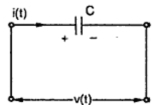### Capacitance

An element in which energy is stored in the form of an electrostatic field is known as capacitance. It is made up of tow conducting plates separated by a dielectric material. It is denoted as 'C' and is measured in farads (F).
The fig shows a capacitor. The voltage across it is time varying v(t) and current through it is also time varying i(t).Fig. 1   Capacitance

Key point : For a capacitor the current through it is proportional to the rate of change of voltage across it.

The constant of proportionality is the capacitance C.

While the ratio of the charge stored to the voltage across the capacitor is known as the capacitance C.

The voltage across the capacitor is given by,

The power in the capacitor is given by,

The energy stored in the capacitor is given by,